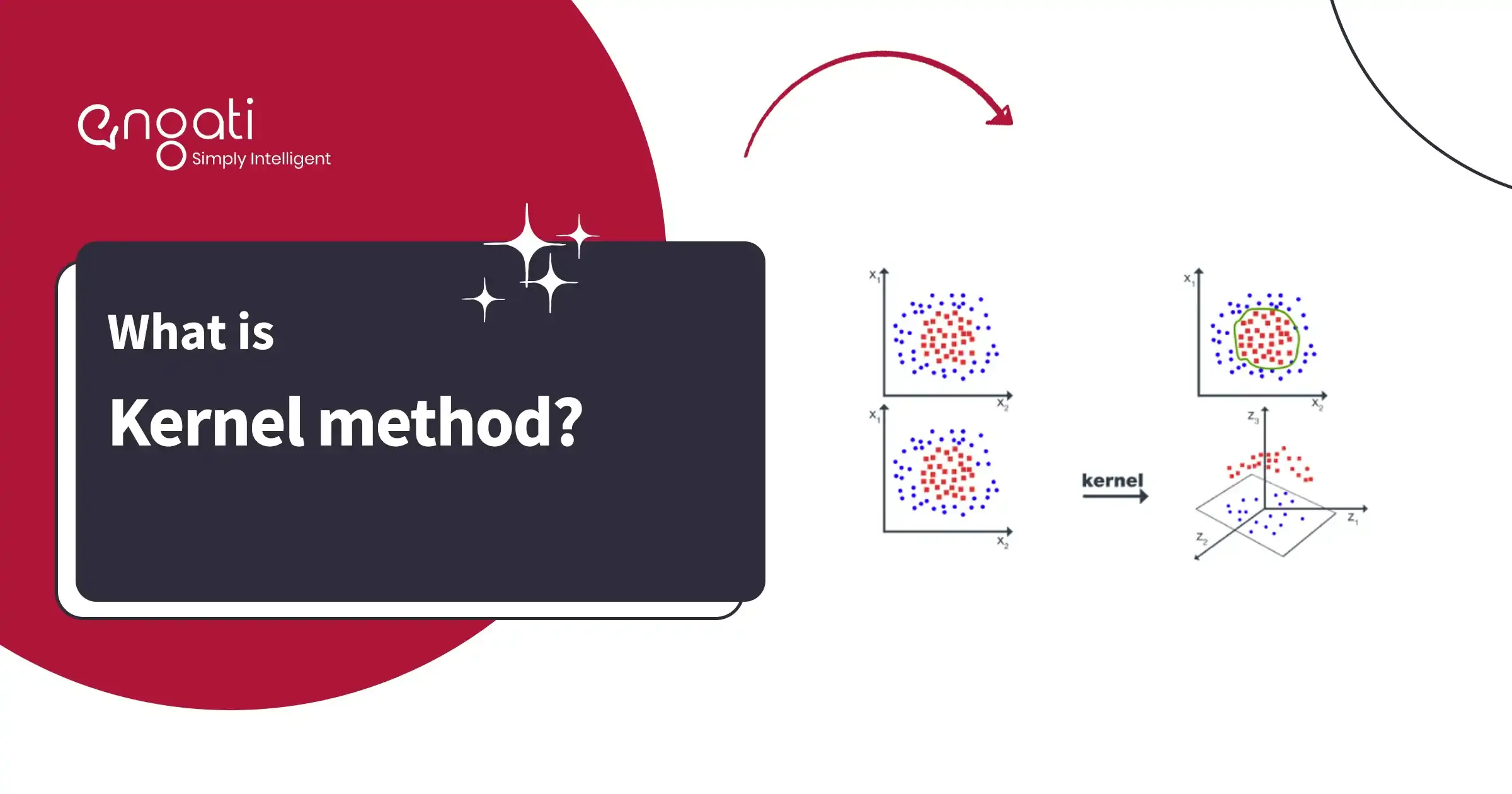# Kernel method## What is Kernel method?

Kernel method is the mathematical technique that is used in machine learning for analyzing data. This method uses Kernel function - that maps data from one space to another space.

It is generally used in Support Vector Machines (SVMs) where the algorithms classify data by finding the hyperplane that separates the data points of different classes.

The most important benefit of Kernel Method is that it can work with non-linearly separable data, and it works with multiple Kernel functions - depending on the type of data.

Because the linear classifier can solve a very limited class of problems, the kernel trick is employed to empower the linear classifier, enabling the SVM to solve a larger class of problems.

## What are the types of Kernel methods in SVM models?

Support vector machines use various kinds of kernel methods. Here are a few of them:

### 1. Linear Kernel

It is used when the data is linearly separable.

K(x1, x2) = x1 . x2

### 2. Polynomial Kernel

It is used when the data is not linearly separable.

K(x1, x2) = (x1 . x2 + 1)d

### 3. Gaussian Kernel

The Gaussian kernel is an example of a radial basis function kernel. It can be represented with this equation:

k(xi, xj) = exp(-𝛾||xi - xj||2)

### 4. Exponential Kernel

Similar to RBF kernel, but it decays much more quickly.

k(x, y) =exp(-||x -y||22)

### 5. Laplacian Kernel

Similar to RBF Kernel, it has a sharper peak and faster decay.

k(x, y) = exp(- ||x - y||)

### 6. Hyperbolic or the Sigmoid Kernel

It is used for non-linear classification problems. It transforms the input data into a higher-dimensional space using the Sigmoid kernel.

k(x, y) = tanh(xTy + c)

### 7. Anova radial basis kernel

It is a multiple-input kernel function that can be used for feature selection.

k(x, y) = k=1nexp(-(xk -yk)2)d

It maps the input data to an to an infinite-dimensional space.

K(x, y) = exp(-γ ||x - y||^2)

### 9. Wavelet kernel

It is a non-stationary kernel function that can be used for time-series analysis.

K(x, y) = ∑φ(i,j) Ψ(x(i),y(j))

### 10. Spectral kernel

This function is based on eigenvalues & eisenvectors of a similarity matrix.

K(x, y) = ∑λi φi(x) φi(y)

### 11. Mahalonibus kernel

This function takes into account the covariance structure of the data.

K(x, y) = exp(-1/2 (x - y)T S^-1 (x - y))

## How Kernel method works?

Kernel method in SVM works in the following way:

1. Data Preprocessing - Cleaning the data & normalizing it for getting the features at the same level.

2. Kernel Function Selection - Select the correct kernel function that maps the data into a higher-dimensional space.

3. Kernel Matrix Computation - Compute the kernel matrix that measures the similarity between the data pointers in the input space.

4. Feature Space Mapping - The kernel matrix is then used to map that input data into the higher-dimensional space.

5. Model Training - The final step is to train the model on the mapped data.

## What is the role of Kernel Method in Support Vector Machines (SVMs)?

SVMs are popular type of machine learning algorithms that are used with kernel method for solving classification & regression problems.

You can break SVM strategy down into two steps:

1. The data is projected implicitly onto a high-dimensional space through the kernel trick.

2. Apply a linear classifier to the projected data.

## How to Choose the Right Kernel Function in SVM?

The choice of kernel in SVM depends on the type of data being analyzed & the problem being solved. Here's how you can go about choosing the right kernel function:

1. Understand the problem - Understand the type of data, features, and the complexity of the relationship between the features

2. Choose a simple kernel function - Start with the linear kernel function as it serves as the baseline for comparison with the complex kernel functions.

3. Test different kernel functions - Test polynomial, RBF kernel, etc and compare their performance.

4. Tune the parameters - Experiment with different parameter values & choose the values that deliver the best performance.

5. Use domain knowledge - Based on the type of data, use the domain knowledge & choose the right type of kernel for your data set.

6. Consider computational complexity - Calculate the computation type & the resources that would be required for larger data sets.

## What are the applications of kernel method in SVM?

1. Classification
2. Regression
3. Clustering
4. Anomaly detection
5. Feature extraction
6. Natural language processing
7. Computer vision
8. Time series analysis
9. Recommender systems
10. Bio-informatics
11. Signal processing
12. RoboticsRequest a Demo!
Get started on Engati with the help of a personalised demo.
This is some text inside of a div block.
This is some text inside of a div block.
This is some text inside of a div block.
This is some text inside of a div block.
*only for sharing demo link on WhatsApp
Thanks for the information.
We will be shortly getting in touch with you.
Oops! something went wrong!
For any query reach out to us on contact@engati.comI am looking for a conversational AI engagement solution for the web and other channels.I would like for a conversational AI engagement solution for WhatsApp as the primary channelI am an e-commerce store with Shopify. I am looking for a conversational AI engagement solution for my businessI am looking to partner with Engati to build conversational AI solutions for other businessesYou're a step away from building your Al chatbot

How many customers do you expect to engage in a month?

Less Than 2000

2000-5000

More than 5000Thanks for the information.

We will be shortly getting in touch with you.